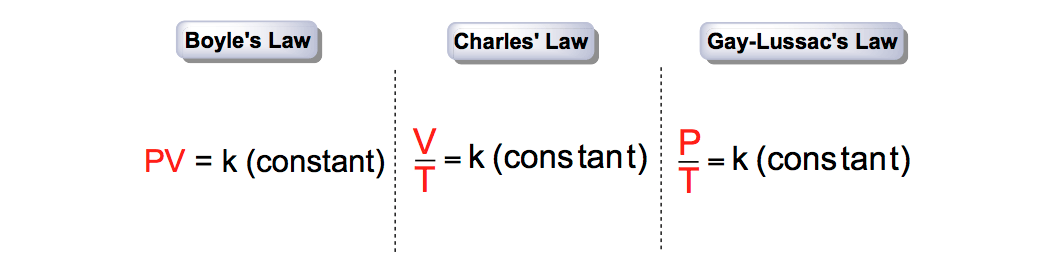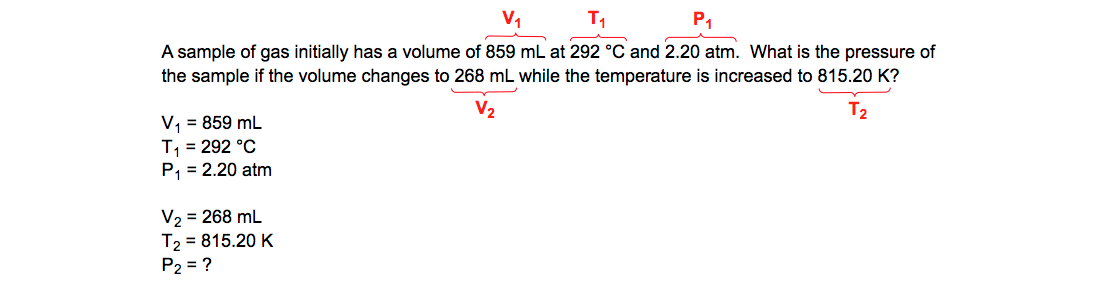Ch.1 - Intro to General Chemistry 2hrs & 53mins 0% complete Worksheet Ch.2 - Atoms & Elements 2hrs & 49mins 0% complete Worksheet Ch.3 - Chemical Reactions 3hrs & 25mins 0% complete Worksheet BONUS: Lab Techniques and Procedures 1hr & 38mins 0% complete Worksheet BONUS: Mathematical Operations and Functions 47mins 0% complete Worksheet Ch.4 - Chemical Quantities & Aqueous Reactions 3hrs & 30mins 0% complete Worksheet Ch.5 - Gases 3hrs & 47mins 0% complete Worksheet Ch.6 - Thermochemistry 2hrs & 28mins 0% complete Worksheet Ch.7 - Quantum Mechanics 2hrs & 35mins 0% complete Worksheet Ch.8 - Periodic Properties of the Elements 1hr & 57mins 0% complete Worksheet Ch.9 - Bonding & Molecular Structure 2hrs & 5mins 0% complete Worksheet Ch.10 - Molecular Shapes & Valence Bond Theory 1hr & 31mins 0% complete Worksheet Ch.11 - Liquids, Solids & Intermolecular Forces 3hrs & 40mins 0% complete Worksheet Ch.12 - Solutions 2hrs & 17mins 0% complete Worksheet Ch.13 - Chemical Kinetics 2hrs & 22mins 0% complete Worksheet Ch.14 - Chemical Equilibrium 2hrs & 26mins 0% complete Worksheet Ch.15 - Acid and Base Equilibrium 4hrs & 42mins 0% complete Worksheet Ch.16 - Aqueous Equilibrium 3hrs & 48mins 0% complete Worksheet Ch. 17 - Chemical Thermodynamics 1hr & 44mins 0% complete Worksheet Ch.18 - Electrochemistry 2hrs & 58mins 0% complete Worksheet Ch.19 - Nuclear Chemistry 1hr & 33mins 0% complete Worksheet Ch.20 - Organic Chemistry 3hrs 0% complete Worksheet Ch.22 - Chemistry of the Nonmetals 2hrs & 1min 0% complete Worksheet Ch.23 - Transition Metals and Coordination Compounds 1hr & 54mins 0% complete Worksheet

# Combined Gas Law

See all sectionsJules Bruno

The Combined Gas Law represents the theorem that explains gas behavior by examining pressure (P), volume (V) and absolute temperature (T) through relating Boyle’s LawCharles’ Law and Gay-Lussac’s Law.

The Simple Gas Laws

The Simple Gas Laws of Boyle’s Law, Charles’ Law and Gay-Lussac’s Law try to explain gas behavior by discussing the variables of pressure (P), volume (V), and absolute temperature (T).

Boyle’s Law

Under Boyle’s Law, pressure (P) and volume (V) are inversely proportional to one another when moles (n) and absolute temperature (T) are held constant. This means as the pressure (P) decreases then the volume (V) increases.

This inverse pressure-volume relationship can be illustrated by the given graph:Pressure-Volume Plot

By isolating the pressure (P) and volume (V) when moles (n) and temperature (T) are held constant gives the simplified Ideal Gas Law expression:Pressure-Volume Expression

Charles’ Law

Charles’ Law examines how the volume of a dry gas either contracts or expands under varying temperatures. According to it, the volume (V) is directly proportional to the absolute temperature (T). This means as the volume (V) increases then the absolute temperature (T) will also increase.

This direct temperature-volume relationship can be illustrated by the given graph:Temperature-Volume Plot

Focusing on the absolute temperature (T) and the volume (V) while maintaining fixed amounts for the pressure (P) and the moles (n) gives:Temperature-Volume Expression

Gay-Lussac’s Law

Under Gay-Lussac’s Law, the pressure (P) of gas molecules is directly proportional to the absolute temperature (T). Pressure represents the amount of force applied to a surface per units of area.Pressure, Force, Area

Increasing the absolute temperature (T) allows gas molecules to absorb this additional thermal energy, which they then convert to kinetic energy. With their greater average kinetic energy the molecules will move faster and collide with the walls of the container with greater force, while the volume remains fixed. This leads to an increase in the pressure (P).

This direct pressure-temperature relationship can be illustrated by the given graph:Pressure-Temperature Plot

Isolating the pressure (P) and absolute temperature (T) while the volume (V) and moles (n) are held constant gives the expression:Pressure-Temperature Expression

Combined Gas Law

The Combined Gas Law examines the connections between pressure (P), volume (V) and absolute temperature (T) when discussing gases. These three variables are related through Boyle’s Law, Charles’ Law and the Gay-Lussac’s Law.Simple Gas Law Expressions

Through the incorporation of these Simple Gas Laws we can isolate the Combined Gas Law as the following expression:Derivation of Combined Gas Law Expression

When using two data sets (2 pressures, 2 volumes & 2 temperatures) you obtain the Combined Gas Law formula as:Combined Gas Law Equation

Now let’s take a look at typical question dealing with the step by step process of using the Combined Gas Law.

PRACTICE: A sample of gas initially has a volume of 859 mL at 292 °C and 2.20 atm.  What is the pressure of the sample if the volume changes to 268 mL while the temperature is increased to 815.20 K?

STEP 1: Identify the given variables.Combined Gas Law (Isolating given variables)

STEP 2: The units for the pressures (P) and volumes (V) can vary as long as they match, but the absolute temperature (T) must always be in Kelvin.Temperature Conversion (Celsius to Kelvin)

STEP 3: Plug the given values into the Combined Gas Law Equation.Combined Gas Law Equation (Solving for P2)

STEP 4: Perform cross-multiplication between the two ratios.Combined Gas Law (Cross Multiplication)

STEP 5: Isolate the missing variable for the second pressure (P2).Combined Gas Law (Isolating P2)

The Combined Gas Law in collaboration with the Simple Gas Laws helps us form the Ideal Gas Law when discussing the behavior of ideal gases under optimal conditions. We can explore the non-ideal behavior of gases when dealing with the Van der Waals Equation, the speed or velocity of gases with the Root Mean Square Equation, the rates of gases with Graham’s Rate of Effusion, and the partial pressures of gases with Dalton’s LawJules Bruno

Jules felt a void in his life after his English degree from Duke, so he started tutoring in 2007 and got a B.S. in Chemistry from FIU. He’s exceptionally skilled at making concepts dead simple and helping students in covalent bonds of knowledge.